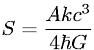Equations > Physics > Modern Physics > Black Hole Entropy as derived by Stephen Hawking

### Black Hole Entropy as derived by Stephen HawkingLatex Code:

MathML Code:

 $S=\frac{\mathrm{Akc}}^{3}4\hslash G$

MathType 5.0: